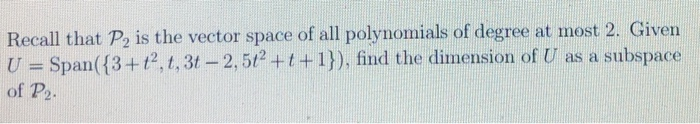# Recall that P2 is the vector space of all polynomials of degree at most 2. Given...

###### Question:Recall that P2 is the vector space of all polynomials of degree at most 2. Given U = Span({3+t?, t, 3t – 2,5t +t+1}), find the dimension of U as a subspace of P2.